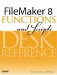# Degrees()

Category: Trigonometric

 Syntax: Degrees ( number )

Parameters:

numberA number representing an angle measured in radians.

Data type returned: Number

Description:

Converts an angle measured in radians to its equivalent in degrees. There are 2xPi radians in 360°, so 1 radian is just over 57°.

Another way to convert radians to degrees is to multiply by 180/Pi.

Examples:

Function

Results

Degrees ( 0 )

Returns 0.

Degrees ( Pi / 4 )

Returns 45.

Degrees ( 2 * Pi )

Returns 360.

Degrees ( 4 * Pi )

Returns 720.

Degrees ( -Pi / 2 )

Returns -90.FileMaker 8 Functions and Scripts Desk Reference
ISBN: 0789735113
EAN: 2147483647
Year: 2004
Pages: 352